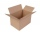# Third power + area - math problems

#### Number of problems found: 37

• Mr DumaMr Duma recently inherited a rectangular plot, part of the estate left by his late father. The plot with the following dimensions: Length=2x+1;Width=x-1. Determine the formula, in terms of x, that best describes the area of the rectangular plot. He has pl
• The length 2The length of a rectangle is 12y2 – 15y + 8 and the width is 7y – 11. Find the area of the rectangle
• Cube-shaped boxThe cube-shaped box is filled to the brim with 2 liters of milk. Calculate the edge and surface of the box.
• Cube V2SThe volume of the cube is 27 dm cubic. Calculate the surface of the cube.
• Cube surfce2volumeCalculate the volume of the cube if its surface is 150 cm2.
• Cuboid to cubeA cuboid with dimensions of 9 cm, 6 cm, and 4 cm has the same volume as a cube. Calculate the surface of this cube.
• EightEight small Christmas balls with a radius of 1 cm have the same volume as one large Christmas ball. What has a bigger surface: eight small balls, or one big ball?
• Magnified cubeIf the lengths of the cube's edges are extended by 5 cm, its volume will increase by 485 cm3. Determine the surface of both the original and the magnified cube.
• Cardboard boxPeter had square cardboard. The length of the pages was an integer in decimetres. He cut four squares with a side of 3 dm from the corners and made a box out of it, which fit exactly 108 cubes with an edge 1 dm long. Julia cut four squares with a side of
• The ballThe ball has a radius of 2m. What percentage of the surface and volume is another sphere whose radius is 20% larger?
• Length of the edgeFind the length of the edge of a cube with a cm2 surface and a volume in cm3 expressed by the same number.
• Right circular coneThe volume of a right circular cone is 5 liters. Calculate the volume of the two parts into which the cone is divided by a plane parallel to the base, one-third of the way down from the vertex to the base.
• Calculate 3Calculate the cube volume whose edge is 3x-1,3x-1,3x-1
• Minimum surfaceFind the length, breadth, and height of the cuboid-shaped box with a minimum surface area, into which 50 cuboid-shaped blocks, each with length, breadth, and height equal to 4 cm, 3 cm, and 2 cm respectively, can be packed.
• Pyramid cutWe cut the regular square pyramid with a parallel plane to the two parts (see figure). The volume of the smaller pyramid is 20% of the volume of the original one. The bottom of the base of the smaller pyramid has a content of 10 cm2. Find the area of the
• Big cubeCalculate the surface of the cube, which is composed of 64 small cubes with an edge 1 cm long.
• Body diagonalThe cuboid has a volume of 32 cm3. Its side surface area is double as one of the square bases. What is the length of the body diagonal?
• CubeOne cube has an edge increased five times. How many times will larger it's surface area and volume?
• Rectangle poolFind dimensions of an open pool with a square bottom with a capacity of 32 m3 to have painted/bricked walls with the least amount of material.
• Area of a cubeCalculate the surface area of a cube if its volume is equal to 729 cubic meters.

Do you have an exciting math question or word problem that you can't solve? Ask a question or post a math problem, and we can try to solve it.

We will send a solution to your e-mail address. Solved examples are also published here. Please enter the e-mail correctly and check whether you don't have a full mailbox.

Third power - math problems. Area - math problems.